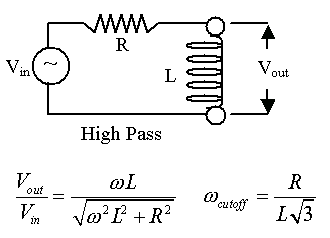### High-Pass Filter in RL-AC Circuits

 Using a resistor (R) and an inductor (L) in series with an AC voltage source, we can create a simple high-pass filter. We by changing the R and L values, you can adjust what frequencies get passed at, say, greater than half the original voltage.     There is an illustration of the circuit below. Set the initial parameters of the circuit : R =  ohms L =    Henries frequency scale factor =            Here are the equations we will use ...